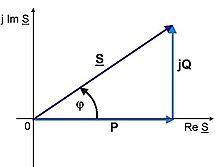# Volt-ampere

Volt-ampere

A volt-ampere (VA) is the unit used for the apparent power in an electrical circuit, equal to the product of root-mean-square (RMS) voltage and RMS current. In direct current (DC) circuits, this product is equal to the real power (active power)  in watts. Volt-amperes are useful only in the context of alternating current (AC) circuits (sinusoidal voltages and currents of the same frequency).Apparent power is the vector sum of real (P) and reactive (jQ) AC power vectors

While both the volt-ampere (abbreviated VA) and the watt have the dimension of power (time rate of energy), they do not have the same meaning. Some devices, including Uninterruptible Power Supplies (UPSs), have ratings both for maximum volt-amperes and maximum watts.

The VA rating is limited by the maximum permissible current, and the watt rating by the power-handling capacity of the device. When a UPS powers equipment which presents a reactive load with a low power factor, neither limit may safely be exceeded.

Wikimedia Foundation. 2010.

### Look at other dictionaries:

• Volt ampere — Volt amp[ e]re (Elec.) A unit of electric measurement equal to the product of a volt and an ampere. For direct current it is a measure of power and is the same as a watt; for alternating current it is a measure of apparent power. [Webster 1913… …   The Collaborative International Dictionary of English

• volt-ampere — [vôlt′am′pir΄] n. Elec. the unit of power equal to one volt times one ampere: in a circuit with direct current it represents power equivalent to one watt: abbrev. VA …   English World dictionary

• volt-ampere — s. m. Nome especial do watt utilizado pela medida de potência aparente de corrente elétrica alternativa (símbolo: VA) …   Dicionário da Língua Portuguesa

• volt-ampere — voltamperas statusas T sritis fizika atitikmenys: angl. volt ampere vok. Voltampere, n rus. вольт ампер, m pranc. voltampère, m …   Fizikos terminų žodynas

• volt-ampere — voltamperas statusas T sritis ekologija ir aplinkotyra apibrėžtis Pilnutinės galios ir reaktyviosios galios matavimo vienetas: V · A. atitikmenys: angl. volt ampere vok. Voltampere, n rus. вольт ампер, m …   Ekologijos terminų aiškinamasis žodynas

• volt-ampere — volt am·pere vōlt am .pi(ə)ralso .pe(ə)r n a unit of electric measurement equal to the product of a volt and an ampere that for direct current constitutes a measure of power equivalent to a watt …   Medical dictionary

• volt-ampere — volt′ am′pere n. elm an electric measurement unit, equal to the product of one volt and one ampere, equivalent to one watt for direct current systems and a unit of apparent power for alternating current systems Abbr.: VA • Etymology: 1895–1900 …   From formal English to slang

• Volt-Ampere-Ohm-Meter — avometras statusas T sritis fizika atitikmenys: angl. avometer; volt ampere ohmmeter; volt ohm ammeter vok. Ampere Volt Ohm Meter, n; Avometer, n; Volt Ampere Ohm Meter, n rus. авометр, m; ампервольтомметр, m; вольтамперомметр, m pranc. ampère… …   Fizikos terminų žodynas

• volt-ampere-ohmmeter — avometras statusas T sritis fizika atitikmenys: angl. avometer; volt ampere ohmmeter; volt ohm ammeter vok. Ampere Volt Ohm Meter, n; Avometer, n; Volt Ampere Ohm Meter, n rus. авометр, m; ампервольтомметр, m; вольтамперомметр, m pranc. ampère… …   Fizikos terminų žodynas

• volt-ampere characteristic — voltamperinė charakteristika statusas T sritis automatika atitikmenys: angl. current voltage characteristic; voltage current characteristic; volt ampere characteristic vok. Stromspannungscharakteristik, f; Strom Spannungs Kennlinie, f;… …   Automatikos terminų žodynas